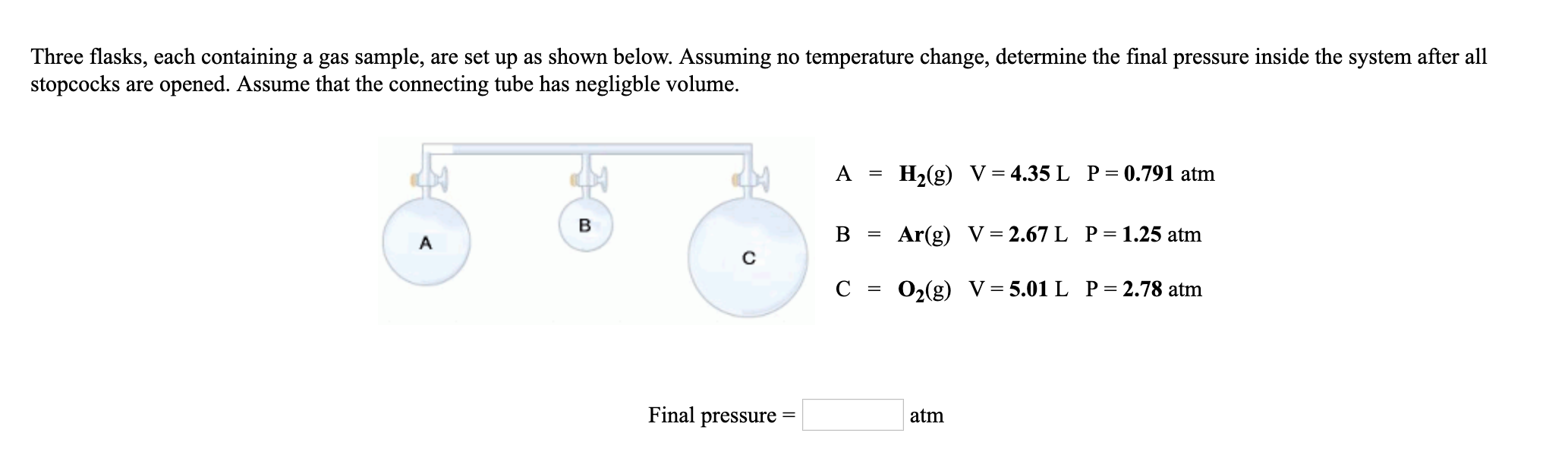# Three flasks, each containing a gas sample, are set up as shown below. Assuming no temperature change, determine the final pressure inside the system after allstopcocks are opened. Assume that the connecting tube has negligble volume.A-H2(g) V-4.35L P-0.791 atmB = Ar(g) V = 2.67 L P= 1.25 atmC = O2(g) V= 5.01 L P= 2.78 atmFinal pressure-atm

Question
31 viewshelp_outlineImage TranscriptioncloseThree flasks, each containing a gas sample, are set up as shown below. Assuming no temperature change, determine the final pressure inside the system after all stopcocks are opened. Assume that the connecting tube has negligble volume. A-H2(g) V-4.35L P-0.791 atm B = Ar(g) V = 2.67 L P= 1.25 atm C = O2(g) V= 5.01 L P= 2.78 atm Final pressure- atm fullscreen
check_circle

Step 1

Total volume of gases can be calculated as

Step 2

Pressure changes can be calculated as (each gases)

Step 3

Final pressure can be...

### Want to see the full answer?

See Solution

#### Want to see this answer and more?

Solutions are written by subject experts who are available 24/7. Questions are typically answered within 1 hour.*

See Solution
*Response times may vary by subject and question.
Tagged in

### Physical Chemistry